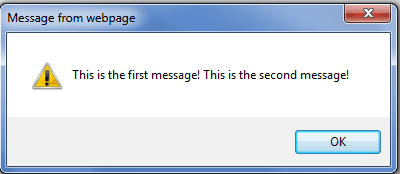# JavaScript ExamplesIn this JavaScript tutorial, we will see various JavaScript examples. I will show you, how to create a function in JavaScript and how we can call that function from a button click. Then we will see how to split a JavaScript string. How to use the JavaScript alert() method? Various JavaScript Confirm box examples. And then we will see how to use if else statement in JavaScript.

## JavaScript Function Example

Let us see what is a JavaScript functions,? How to create a function in JavaScript and then how to call a JavaScript function on a button click.

A function is a block of statements that can perform some task as well it can also return some value. A function is independent of your program and not executed until called.

Functions must be declared before they can be used. Normally functions are placed in the tag of the HTML document to ensure that they are defined before used.

In JavaScript, we can define a function by using the function keyword. Also, a function can take some parameters.

Syntax:

``````function function_name ()
{
statement 1;
statement 2;
}
function function_name (parameter1, parameter 2)
{
statement 1;
statement 2;
}``````

Example:

Here in this example, we write a method inside the tag, which will give an alert message. And in a hyperlink we are calling that method in the body like below:

``````<head runat=”server”>
<title>JavaScript Function Example</title>
<script language=”JavaScript” type=”text/javascript”>
function HelloWord() {
}
</script>
<body>
<form id=”form1″ runat=”server”>
<div>
</div>
</form>
</body>``````

Similarly, if you want to call the javascript method in an asp button control, then you can call the method in the OnClientClick event like below:

``<asp:Button ID="btnCall" runat="server" Text="Call JavaScript Function" OnClientClick="HelloWord();" />``

## Split javascript string example

Now, we will see how to split a JavaScript string.

Here is the string:

``````var strMainString = new String();
strMainString = “AspDotNetHelp.com|SharePointDotNet.com|EnjoySharePoint.com|Fewlines4Biju.com”;``````

Now we can split the string with separator “|” and we will store in an array.

``````var arr = new Array();
arr = myString.split(“|”);
Now we can retrieve the items from the array by using a for loop statement like below:
for(var i=0; i < arr.length; i++)
{
}``````

This will split the string in JavaScript and when you will access using array index, it will display each item.

## Various alert() method examples in JavaScript

Now we will see some examples of alert() method in javascript.

Below are some examples of JavaScript dialog boxes:

### Example-1 (Simple JavaScript Alert Box):

This example we will how we can display a simple javascript alert box.

``````<script language=”JavaScript” type=”text/javascript”>
</script>``````

### Example-2 (Display message in multiple lines):

In this example, we can see how we can show a message in the dialog box in multiple lines.

``````<script language=”JavaScript” type=”text/javascript”>
</script>``````

### Example-3 (Show Messages from variables in dialog box):

In this example, we will see how we can show message from variables in a dialog box.

``````<script language=”JavaScript” type=”text/javascript”>
var firstmsg = “This is the first message! “;
var secondmsg = “This is the second message! “;
</script>``````

## JavaScript Confirm box examples

Now, we will see how we can show confirmation box in JavaScript, few JavaScript Confirm box examples.

The confirm dialog box is used to confirm a user’s answer to a question. A question mark will appear in the box with an OK button and a Cancel button. If the user presses the OK button, true is returned; if he presses the Cancel button, false is returned.

Example (JavaScript Confirm box):

``````<script language=”JavaScript” type=”text/javascript”>
if (confirm(“Are you sure you want to do this?”) == true) {
}
else {
}
</script>``````

When run this, the window will appear like below:

If you click on OK, the alert will come like below:

And if you click on Cancel, the alert will come like below

## If Else statements Example in JavaScript

Now, we will discuss how to use if else statement in javascript.

Syntax of if else statement:

``````if (condition) {
statements1;
}
else if (condition)  {
statements2;
}
else if (condition)  {
statements3;
}
else{
statements4;
}``````

Example:

Below is an example of if else statement:

``````<script language=”JavaScript” type=”text/javascript”>
var age = eval(prompt(“How much you scored?”, “”));
if (age >= 60) {
}
else if (age > 50 && age < 60) {
}
else if (age >= 30 && age < 50) {
}
else {
}
</script>``````

Here in this example, it will first prompt the user to enter a value and according to the condition, it will alert the message. See fig below:

Once you enter the value it will show alert box according to the condition like below:

You may like following JavaScript tutorials:

## Conclusion

Here we saw various JavaScript examples. We saw how to create and call a JavaScript function on a button click. How to use If Else statements in JavaScript, Confirm box example in JavaScript, alert() method examples in JavaScript, Split javascript string.

Check out Best Alternative to InfoPath -> Try NowSharePoint Online FREE Training

JOIN a FREE SharePoint Video Course (3 Part Video Series)